# High School Chemistry : Electrochemistry

## Example Questions

← Previous 1 3

### Example Question #1 : Electrochemistry

Which statement is true of a galvanic cell?

Electrons travel from anode to cathode, oxidation occurs at the cathode, the cathode is the negative electrode, and the reaction is spontaneous

Electrons travel from anode to cathode, reduction occurs at the cathode, the anode is the negative electrode, and the reaction is spontaneous

Electrons travel from cathode to anode, reduction occurs at the anode, the cathode is the negative electrode, and the reaction is nonspontaneous

Electrons travel from cathode to anode, oxidation occurs at the anode, the anode is the negative electrode, and the reaction is spontaneous

Electrons travel from anode to cathode, reduction occurs at the cathode, the anode is the negative electrode, and the reaction is nonspontaneous

Electrons travel from anode to cathode, reduction occurs at the cathode, the anode is the negative electrode, and the reaction is spontaneous

Explanation:

Galvanic cells always involve spontanous oxidation-reduction reactions. In any electrochemical cell, the electrons always move from anode to cathode. Also, the anode is always the site of oxidation, and the cathode is always the site of reduction. Since the reaction is spontaneous (net release of free energy) it drives the movement of electrons from the anode to the cathode. Remember, oxidation is loss of electrons and reduction is gain of electrons. Since oxidation always occurs at the anode, we are left with an excess of electrons, making it the negative electrode. It should makes sense that the extra electrons from the anode spontaneously travel to the cathode (positive electrode).

To help remember oxidation-reduction processes, consider the mnemonics "OIL RIG" and "An Ox, Red Cat." OIL RIG stands for "oxidation is loss, reduction is gain" in reference to electrons. An Ox, Red Cat tells us that the anode is the site of oxidation, while the cathode is the site of reduction.

### Example Question #1 : Electrochemistry

Which of the following differences between galvanic cells and electrolytic cells is false?

Electrolytic cells have oxidation take place at the cathode

Electrolytic cells are non-spontaneous

Electrolytic cells have a positive Gibb's free energy

Electrolytic cells have negative voltages

Electrolytic cells have oxidation take place at the cathode

Explanation:

Electrolytic cells use non-spontaneous reactions that require an external power source in order to proceed. The values between galvanic and electrolytic cells are opposite of one another. Galvanic cells have positive voltage potentials, while electrolytic voltage potentials are negative. Both types of cell, however, have oxidation occur at the cathode and reduction occur at the anode.

### Example Question #2 : Help With Galvanic Cells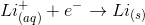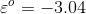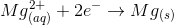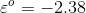For the following reaction to occur does the does the electrochemical cell voltaic or galvanic?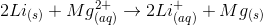Neither

Electrolytic

Both

Galvanic

Galvanic

Explanation:

First we must rearrange the reduction potentials so that when added together, they match the reaction that takes place in the electrochemical cell.In the overall reaction,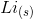is in the reactant side, so theequation must be inverted.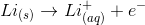Use the equation: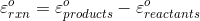to find the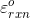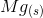is product, whileis the reactant.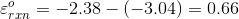The cell must be galvanic because thevalue is positive. This means, this the reaction is a spontaneous reaction occurs without an outside energy source.

### Example Question #1 : Electrochemistry

How does an electrolytic cell differ from a galvanic cell?

The cell potential is positive in an electrolytic cell

Oxidation takes place at the anode in an electrolytic cell, but at the cathode in a galvanic cell

Oxidation takes place at the anode in a galvanic cell, but at the cathode in an electrolytic cell

The cathode is marked negative in an electrolytic cell

The cathode is marked negative in an electrolytic cell

Explanation:

Oxidation always takes place at the anode, regardless of the electrical cell type. The charges on the anode and cathode are reversed between galvanic and electrolytic cells. In electrolytic cells, the cathodes are marked negative and the anodes are marked positive. In galvanic cells, the reverse is true: cathodes are marked positive and anodes are marked negative.

### Example Question #143 : Thermochemistry And Kinetics

Toward which pole do the electrons travel in an electrolytic cell?

Towards the positive pole

The electrons do not travel; only the protons travel

Towards the anode

Towards the cathode

Towards the cathode

Explanation:

Reduction always occurs at the cathode, and oxidation always occurs at the anode. Since reduction is the addition of electrons, electrons must travel toward the site of reduction.

In an electrolytic cell the negative charge is on the cathode, while the positive charge is on the anode. Since an electrolytic cell requires energy to perpetuate the reaction, we are pushing the electrons against their potential gradient. The electrons, which are negatively charged, are traveling towards the cathode, which is also negatively charged.

### Example Question #1 : Electrochemistry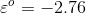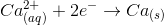The following reaction below takes place in an electrochemical cell: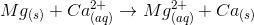Is the cell galvanic or voltaic?

Neither

Electrolytic

Both

Galvanic

Galvanic

Explanation:Since our overall reaction includes magnesium solid in the reactants, we must invert the first equation, including the sign of the electrical potential.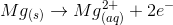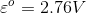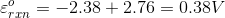This cell is galvanic because the electrical potential,, is positive. Galvanic cells involve spontaneous reactions, and therefore, do not need any external energy source to drive the reaction.

### Example Question #1 : Help With Electrolytic Cells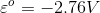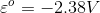The following reaction below takes place in an electrochemical cell: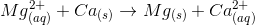Which of the following best describes this cell?

Galvanic

Neither

Electrolytic

Both

Electrolytic

Explanation:Since our overall reaction includes calcium solid in the reactants, we must invert the second equation, including the sign of the electrical potential.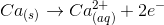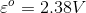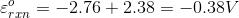This cell is electrolytic because the electrical potential,, is negative. Electrolytic cells involve nonspontaneous reactions, and therefore, must have an external voltage source such as a battery to drive their reactions.

### Example Question #2 : Electrochemistry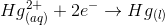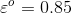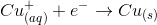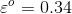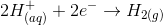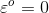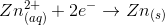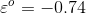Which of the following species would mostly likely be oxidized, if placed in a electrochemical cell with another species?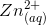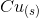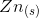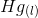Explanation:

Using the equation:, for a spontaneous reaction to occur,must be positive. With solid zinc as the reactant, any other solid can act as the product, and still give a positive. This is because, subtracting a negative number will give a positive value.

As a result, the equation,, will become inverted to make the solid zinc a reactant.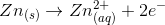. Solid zinc will give electrons, and oxidize, to reduce other ions.

### Example Question #5 : ElectrochemistryFor the following reaction to occur does the does the electrochemical cell voltaic or galvanic?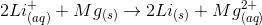Neither

Electrolytic

Galvanic

Both

Electrolytic

Explanation:

First we must rearrange the reduction potentials so that when added together, they match the reaction that takes place in the electrochemical cell.In the overall reaction,is in the reactant side, so theequation must be inverted.Use the equation:to find theis product, whileis the reactant.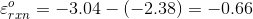The cell must be electrolytic because thevalue is negative. This means, this the reaction is a non-spontaneous reaction and need a applied energy source to make the reaction take place.

### Example Question #6 : Electrochemistry

How does an electrolytic cell differ from a galvanic cell?

Oxidation takes place at the anode in an electrolytic cell, but at the cathode in a galvanic cell

The cathode is marked negative in an electrolytic cell

The cell potential is positive in an electrolytic cell

Oxidation takes place at the anode in a galvanic cell, but at the cathode in an electrolytic cell